# Mobius strip

## Definition

### Verbal definition

The infinite Mobius stripor open Mobius strip is obtained by taking an infinite rectangular strip and identifying the opposite sides of the rectangular strip, with opposite orientations.

For the closed Mobius strip, we replace the infinite rectangular strip with a rectangular strip of finite height.

### Coordinate definition

The infinite Mobius strip or open Mobius strip is the quotient of the rectangular strip$|x| \le 1$ in the real Euclidean plane, by the relation: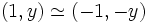$(1,y) \simeq (-1,-y)$

### Alternate descriptions

The Mobius strip is equivalent to the set of all undirected lines in the plane. To achieve this identification, fix an porigin and a choice of coordinate axes. Now, for any line in the plane, associate the following two quantities: its direction, and the signed distance from the origin. The direction can be sent t othe$x$-coordinate in the Mobius strip and the signed distance, to the$y$-coordinate.

## Application of constructs

### Compactifications

• The one-point compactification of the Mobius strip is the real projective plane
• If we view the Mobius strip as a fibre bundle over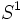$S^1$ with fibres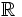$\R$, then the fibre-wise compactification is the Klein bottle (this is a fibre bundle of$S^1$ over$S^1$).

### Covering spaces

The cylinder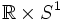$\R \times S^1$ is a double cover of the Mobius strip.

The Mobius strip is set-theoretically a direct product of$\R$ and the projective plane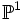$\mathbb{P}^1$ (which is the same as$S^1$). However, it is not topologically a direct product. Intuitively we can explain this as follows -- the identification of the two ends of the strip with opposite orientations means that one can start with a point, and keep going around with the same$y$-coordinate locally to end up at a point whose$y$-coordinate is the negative of the original.

However, if we go around twice, we end up at the original point. This is essentially because the double cover of the Mobius strip is$\R \times S^1$.

## Invariants

### Fundamental group

The fundamental group of the Mobius strip is$\mathbb{Z}$. The generator for this is the loop that goes once around the Mobius strip.

Further information: Fundamental group of Mobius strip

### Homology

Further information: homology of Mobius strip